NCERT Solutions for Class 8 Maths Chapter 2 Linear Equations in One Variable Ex 2.4 are part of NCERT Solutions for Class 8 Maths. Here we have given NCERT Solutions for Class 8 Maths Chapter 2 Linear Equations in One Variable Ex 2.4.

 Board CBSE Textbook NCERT Class Class 8 Subject Maths Chapter Chapter 2 Chapter Name Linear Equations in One Variable Exercise Ex 2.4 Number of Questions Solved 10 Category NCERT Solutions

## NCERT Solutions for Class 8 Maths Chapter 2 Linear Equations in One Variable Ex 2.4

Question 1.
Amina thinks of a number and subtracts $$\frac { 5 }{ 2 }$$ from it. She multiplies the result by 8. The result now obtained is 3 times the same number she thought of. What is the number?
Solution.
Let the number be x.
Then, according to the question,Question 2.
A positive number is 5 times another number. If 21 is added to both the numbers, then one of the new numbers becomes twice the other new number. What are the numbers?
Solution.
Let the numbers be x and 5x.
If 21 is added to both the numbers, then first new number = x + 21
and, second new number = 5x + 21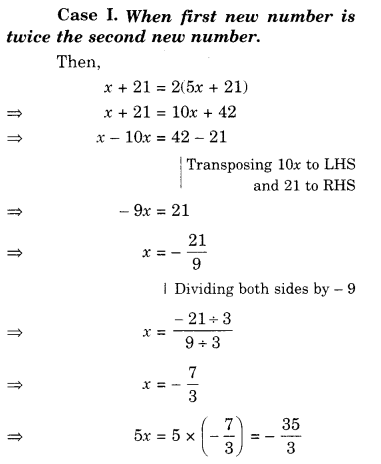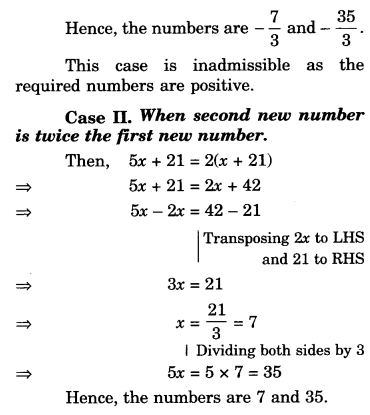Question 3.
Sum of the digits of a two-digit number is 9. When we interchange the digits, it is found that the resulting new number is greater than the original number by 27. What is the two-digit number?
Solution.
Let the unit’s digit of the two-digit number be x.
Then, the ten’s digit of the two-digit number = 9 – x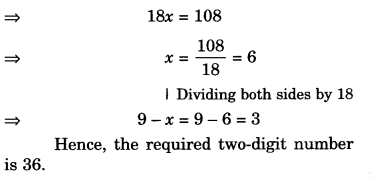Question 4.
One of the two digits of a two digit number is three times the other digit. If you interchange the digits of this two-digit number and add the resulting number to the original number, you get 88. What is the original number?
Solution.
Let in the original numberQuestion 5.
Shobo’s mother’s present age is six times Shobo’s present age. Shobo’s age five years from now will be one third of his mother’s present age. What are their present ages?
Solution.
Let the present age of Shobo be x  year. Then, the present age of Shobo’s mother = 6x years.
Five years from now
Shobo’s age = (x + 5) years
According to the question,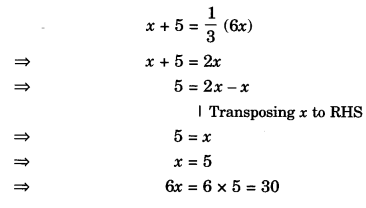Hence, their present ages are 5 years and 30 years.

Question 6.
There is a narrow rectangular plot, reserved for a school, in Mahuli village. The length and breadth of the plot are in the ratio 11:4. At the rate of ₹ 100 per meter, it will cost the village panchayat ₹ 75000 to fence the plot. What are the dimensions of the plot?
Solution.
Let the length and breadth of the plot be 11x m and 4x m respectively.
Then, the perimeter of the plot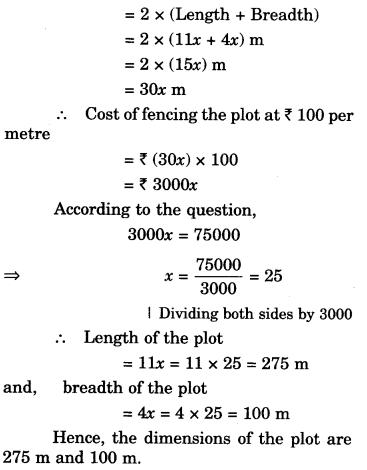Question 7.
Hasan buys two kinds of cloth materials for school uniforms, shirt material that costs him ₹ 50 per meter and trouser material that costs him ₹ 90 per meter. For every 2 meters of the trouser material, he buys 3 meters of the shirt material. He sells the materials at 12% and 10% profit respectively. His total sale is ₹ 36,600. How much trouser material did he buy?
Solution.
Suppose that he bought x meters of trouser material.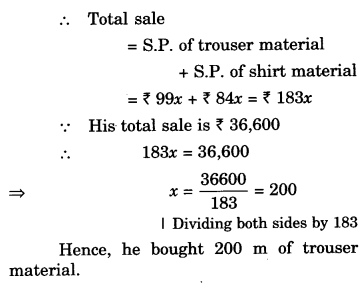Question 8.
Half of a herd of deer are grazing in the field and three fourths of the remaining are playing nearby. The rest 9 are drinking water from the pond. Find the number of deer in the herd.
Solution.
Let the number of deer in the herd be x.Question 9.
A grandfather is ten times older than his granddaughter. He is also 54 years older than her. Find their present ages.
Solution.
Let the present age of granddaughter be x years
Then, the present age of grandfather is 10x years
According to the question,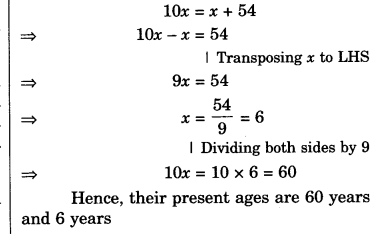Question 10.
Am.an’s age is three times his son’s age. Ten years ago he was five times his son’s age. Find their present ages.
Solution.
Let the present age of Aman’s son be x years
Then, the present age of Aman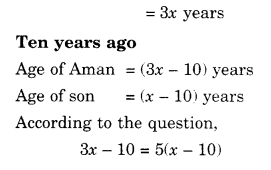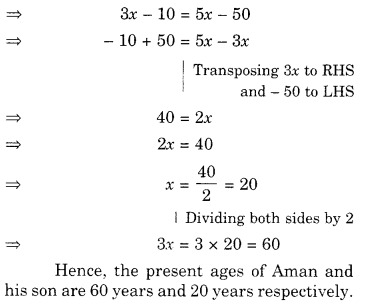We hope the NCERT Solutions for Class 8 Maths Chapter 2 Linear Equations in One Variable Ex 2.4 help you. If you have any query regarding NCERT Solutions for Class 8 Maths Chapter 2 Linear Equations in One Variable Ex 2.4, drop a comment below and we will get back to you at the earliest.